# Andrew Ng机器学习编程作业:Anomaly Detection and Recommender Systems

2018/12/19 21:46

machine-learning-ex8

# 1. 异常检测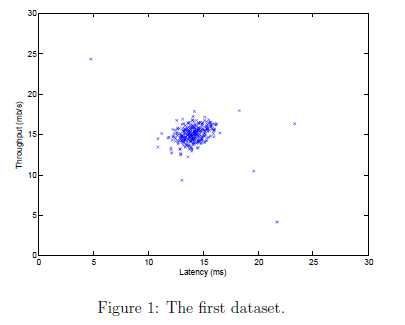## 1.1 高斯分布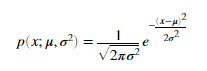## 1.2 估计高斯的参数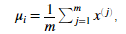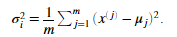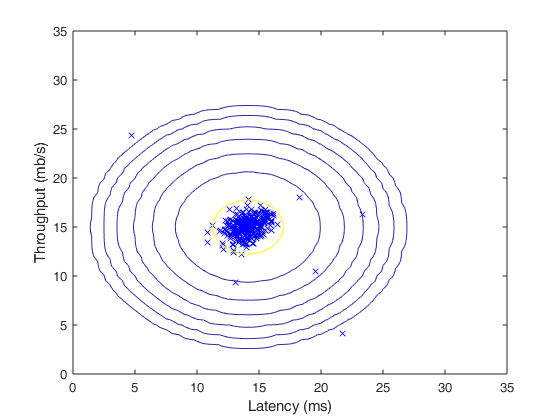estimateGaussian.m内代码

mu = mean(X);

sigma2 = mean((X-mu).^2)';

mu = mu';

## 1.3 选择阈值ε

selectThreshold.m函数应该返回两个值。第一个是阈值ε，如果一个样本x的概率小于ε，那它将被认为成异样样本。函数应该同样返回F1score，告诉我们我们的阈值找的有多么好。对于不同的ε我们将计算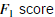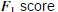通过如下公式计算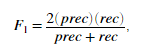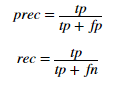• P：精确率是针对我们预测结果而言的，它表示的是预测为正的样本中有多少是真正的正样本。那么预测为正就有两种可能了，一种就是把正类预测为正类(TP)，另一种就是把负类预测为正类(FP)，也就是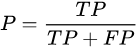• R：而召回率是针对我们原来的样本而言的，它表示的是样本中的正例有多少被预测正确了。那也有两种可能，一种是把原来的正类预测成正类(TP)，另一种就是把原来的正类预测为负类(FN)。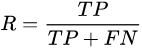selectThreshold.m的代码：

predictions = (pval<epsilon);

tp = sum((predictions==1)&(yval==1));
fp = sum((predictions==1)&(yval==0));
fn = sum((predictions==0)&(yval==1));

prec = tp/(tp+fp);
rec = tp/(tp+fn);
F1 = 2*prec*rec/(prec+rec);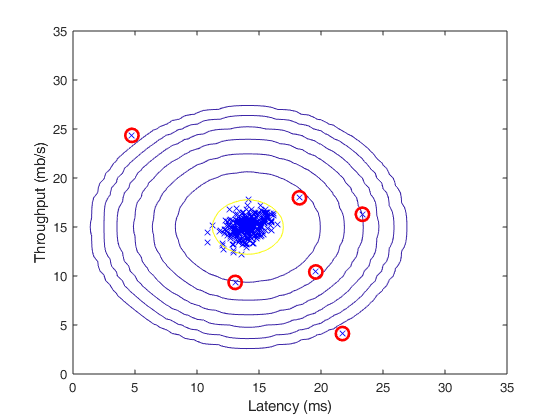## 1.4 高维度数据集

%  Loads the second dataset. You should now have the variables X, Xval, yval in your environment

%  Apply the same steps to the larger dataset
[mu, sigma2] = estimateGaussian(X);

%  Training set
p = multivariateGaussian(X, mu, sigma2);

%  Cross-validation set
pval = multivariateGaussian(Xval, mu, sigma2);

%  Find the best threshold
[epsilon, F1] = selectThreshold(yval, pval);
fprintf('Best epsilon found using cross-validation: %e\n', epsilon);
fprintf('Best F1 on Cross Validation Set:  %f\n', F1);
fprintf('# Outliers found: %d\n', sum(p < epsilon));

# 2 推荐系统

## 2.1 电影评分数据集

load('ex8_movies.mat');
Y is a 1682 x 943 matrix, containing ratings (1 - 5) of 1682 movies on 943 users
R is a 1682 x 943 matrix, where R(i,j) = 1 if and only if user j gave a rating to movie i
% From the matrix, we can compute statistics like average rating.
fprintf('Average rating for movie 1 (Toy Story): %f / 5\n\n', mean(Y(1, R(1, :))));

imagesc(Y);
ylabel('Movies');
xlabel('Users');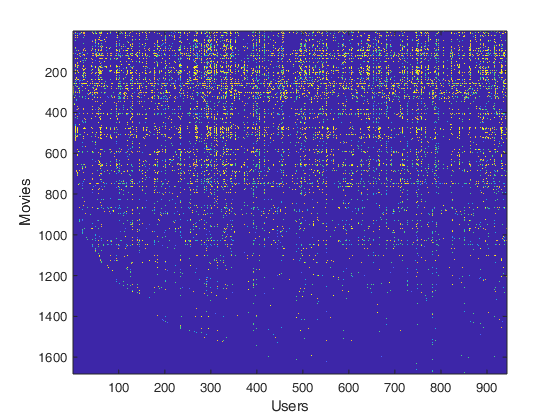## 2.2 协同过滤算法

### 2.2.1 协同过滤的代价函数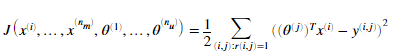cofiCostFunc.m代码

J = 1/2*sum(sum(((X*Theta'-Y).*R).^2));

### 2.2.2 协同过滤算法的梯度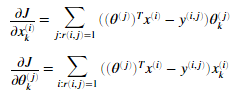当我们完成后，梯度检测将会检测我们的梯度。如果梯度正确我们应该看到产生与梯度检测相近的结果。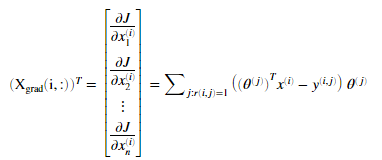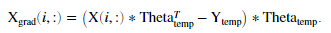cofiCostFunc.m代码

J = 1/2*sum(sum(((X*Theta'-Y).*R).^2));

Theta_grad = ((Theta*X'-Y').*R')*X;

### 2.2.3 正则化代价函数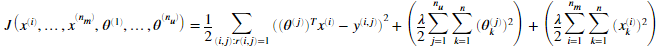完成 cofiCostFunc.m的代码

J = 1/2*sum(sum(((X*Theta'-Y).*R).^2));

J = J+lambda/2*sum(sum(X.^2));
J = J+lambda/2*sum(sum(Theta.^2));

### 2.2.4 正则化梯度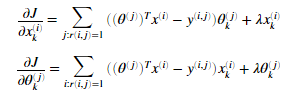cofiCostFunc.m内代码

J = 1/2*sum(sum(((X*Theta'-Y).*R).^2));

J = J+lambda/2*sum(sum(X.^2));
J = J+lambda/2*sum(sum(Theta.^2));

Theta_grad = Theta_grad+lambda*Theta;

## 2.3 学习电影推荐系统

% Load movvie list

% Initialize my ratings
my_ratings = zeros(1682, 1);

% Check the file movie_idx.txt for id of each movie in our dataset
% For example, Toy Story (1995) has ID 1, so to rate it "4", you can set
my_ratings(1) = 4;
% Or suppose did not enjoy Silence of the Lambs (1991), you can set
my_ratings(98) = 2;

% We have selected a few movies we liked / did not like and the ratings we gave are as follows:
my_ratings(7) = 3;
my_ratings(12)= 5;
my_ratings(54) = 4;
my_ratings(64)= 5;
my_ratings(66)= 3;
my_ratings(69) = 5;
my_ratings(183) = 4;
my_ratings(226) = 5;
my_ratings(355)= 5;

fprintf('\n\nNew user ratings:\n');
for i = 1:length(my_ratings)
if my_ratings(i) > 0
fprintf('Rated %d for %s\n', my_ratings(i), movieList{i});
end
end

### 2.3.1 推荐系统

%  Load data

%  Y is a 1682x943 matrix, containing ratings (1-5) of 1682 movies by 943 users
%  R is a 1682x943 matrix, where R(i,j) = 1 if and only if user j gave a rating to movie i
%  Add our own ratings to the data matrix
Y = [my_ratings Y];
R = [(my_ratings ~= 0) R];

%  Normalize Ratings
[Ynorm, Ymean] = normalizeRatings(Y, R);

%  Useful Values
num_users = size(Y, 2);
num_movies = size(Y, 1);
num_features = 10;

% Set Initial Parameters (Theta, X)
X = randn(num_movies, num_features);
Theta = randn(num_users, num_features);
initial_parameters = [X(:); Theta(:)];

% Set options for fmincg

% Set Regularization
lambda = 10;
theta = fmincg(@(t)(cofiCostFunc(t, Y, R, num_users, num_movies, num_features,lambda)), initial_parameters, options);

% Unfold the returned theta back into U and W
X = reshape(theta(1:num_movies*num_features), num_movies, num_features);
Theta = reshape(theta(num_movies*num_features+1:end), num_users, num_features);p = X * Theta';
my_predictions = p(:,1) + Ymean;

[r, ix] = sort(my_predictions,'descend');
for i=1:10
j = ix(i);
if i == 1
fprintf('\nTop recommendations for you:\n');
end
fprintf('Predicting rating %.1f for movie %s\n', my_predictions(j), movieList{j});
end
for i = 1:length(my_ratings)
if i == 1
fprintf('\n\nOriginal ratings provided:\n');
end
if my_ratings(i) > 0
fprintf('Rated %d for %s\n', my_ratings(i), movieList{i});
end### 作者的其它热门文章

0
0 收藏

0 评论
0 收藏
0